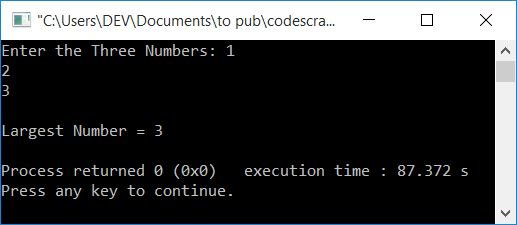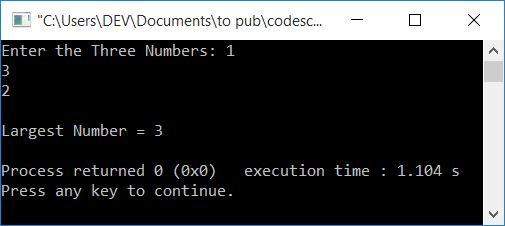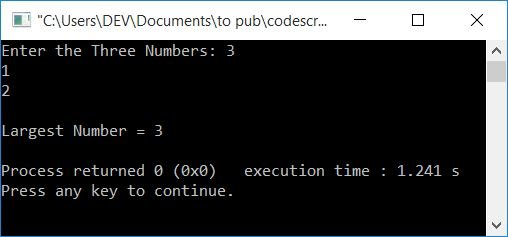# C++ Program to Find the Largest of Three Numbers

This article will teach you how to use C++ to find and print the largest of three numbers entered by the user at run-time. The program is created in the following ways:

Both programs uses if-else to find and print the largest or biggest among any three numbers entered by the user.

## In C++, find the largest of three numbers

To find the largest number among three numbers in C++ programming, you have to ask the user to enter the three numbers. Now use the if-else ladder to find out which of the three is the largest, as shown in the program given below:

The question is, "Write a program in C++ that finds the largest of three numbers." Here is its answer:

```#include<iostream>
using namespace std;
int main()
{
int numOne, numTwo, numThree, larg;
cout<<"Enter the Three Numbers: ";
cin>>numOne>>numTwo>>numThree;
if(numOne>numTwo)
{
if(numTwo>numThree)
larg = numOne;
else
{
if(numThree>numOne)
larg = numThree;
else
larg = numOne;
}
}
else
{
if(numTwo>numThree)
larg = numTwo;
else
larg = numThree;
}
cout<<"\nLargest Number = "<<larg;
cout<<endl;
return 0;
}```

This program was built and runs under the Code::Blocks IDE. Here is its sample run:Now supply any three numbers as input, say 1, 2, and 3. The third number is the largest in this case, and the second number is the second-largest. Press the ENTER key to print the largest of these three numbers, as shown in the output given below:Here is another sample run with user inputs 1, 3, and 2. The second number is the largest in this case, and the third number is the second largest:Here is one more sample run with user input: 3, 1, 2:#### Main Logic of the Previous Program

The main logic behind the code is:

• Initially, it checks whether the first number is greater than the second:
• If the first number is greater than the second, then check whether the second number is greater than the third:
• if it is true. Then the first number exceeds the second, and the second number exceeds the third.
• So the first number is the largest number.
• If the second number is not greater than the third, the third number is greater than the second.
• Because the third number is greater than the second, check whether the third number is also greater than the first:
• if it is true. The third number is then greater than the first and second.
• So the third number is the largest number.
• If the third number is not greater than the first, the first number is greater than the third.
• Because the first number is greater than both the third and second
• So the first number is the largest number.
• If the first number is not greater than the second, then the second number is greater than the first:
• Because the second number is greater than the first, I checked whether the second number is also greater than the third:
• if it is true. The second number is then greater than both the first and third numbers.
• So the second number is the largest.
• If the second number is not greater than the third, the third number is greater than the second.
• because the third number is higher than the second, and the second number is higher than the first.
• So the third number is the largest.

#### The previous program is demonstrated with a dry run

The dry run of the above program with user inputs 2, 1, and 3 goes like this:

• When the user enters 2, 1, and 3 in the same pattern, then 2 gets stored in numOne, 1 gets stored in numTwo, and 3 gets stored in numThree. That is, numOne=2, numTwo=1, and numThree=3.
• Now that the condition numOne>numTwo or 2>1 evaluates to be true, program flow goes inside the if block.
• Now the condition numTwo>numThree or 1>3 evaluates to be false, so the program flow does not go inside if's body, but rather to the else's body.
• There, the condition numThree>numOne or 3>2 evaluates to true, program flow goes inside the if's body.
• And the value of numThree is set to larg.
• The program flow will skip else's part because the condition evaluates to true.
• And because the outermost if-else condition evaluates to true, else's part is skipped in this case as well.
• So program flow goes to the statement that prints the value of larg as output.
• That will print the largest among the three numbers entered.

## Using a C++ function, find the largest of three numbers

This program does the same job as the previous program. But internally, this program uses a different approach to do the task. That is, this program is created using a user-defined function, findLargest().

The function findLargest() takes three numbers as its arguments and returns the largest among them. So the largest number gets returned by this function and initialized to larg inside the main() function. Therefore, just print the value of larg.

```#include<iostream>
using namespace std;
int findLargest(int, int, int);
int main()
{
int numOne, numTwo, numThree, larg;
cout<<"Enter the Three Numbers: ";
cin>>numOne>>numTwo>>numThree;
larg = findLargest(numOne, numTwo, numThree);
cout<<"\nLargest Number = "<<larg;
cout<<endl;
return 0;
}
int findLargest(int nOne, int nTwo, int nThree)
{
if(nOne>nTwo)
{
if(nTwo>nThree)
return nOne;
else
{
if(nThree>nOne)
return nThree;
else
return nOne;
}
}
else
{
if(nTwo>nThree)
return nTwo;
else
return nThree;
}
}```

This program produces the same output as the previous program.

#### The same program in different languages

C++ Quiz

« Previous Program Next Program »